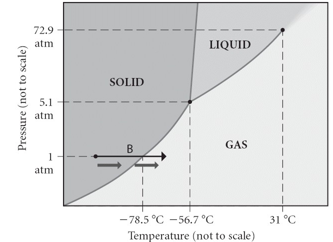# Problem: Consider the phase diagram shown.  Choose the statement below that is TRUE.   A) The triple point of this substance occurs at a temperature of 31°C. B) At 10 atm of pressure, there is no temperature where the liquid phase of this substance would exist. C) The solid phase of this substance is higher in density than the liquid phase. D) The line separating the solid and liquid phases represents the ΔHvap. E) None of the above are true.

###### FREE Expert Solution
99% (145 ratings)
###### Problem Details

Consider the phase diagram shown.  Choose the statement below that is TRUE.

A) The triple point of this substance occurs at a temperature of 31°C.

B) At 10 atm of pressure, there is no temperature where the liquid phase of this substance would exist.

C) The solid phase of this substance is higher in density than the liquid phase.

D) The line separating the solid and liquid phases represents the ΔHvap.

E) None of the above are true.What scientific concept do you need to know in order to solve this problem?

Our tutors have indicated that to solve this problem you will need to apply the Phase Diagram concept. You can view video lessons to learn Phase Diagram. Or if you need more Phase Diagram practice, you can also practice Phase Diagram practice problems.

What is the difficulty of this problem?

Our tutors rated the difficulty ofConsider the phase diagram shown.  Choose the statement belo...as low difficulty.

How long does this problem take to solve?

Our expert Chemistry tutor, Jules took 2 minutes and 27 seconds to solve this problem. You can follow their steps in the video explanation above.

What professor is this problem relevant for?

Based on our data, we think this problem is relevant for Professor Ley's class at MDC.